# CSE 313 Data Communication Lec 03 Sabbir Muhammad

• Slides: 15
Download presentationCSE 313 Data Communication Lec 03 Sabbir Muhammad Saleh 1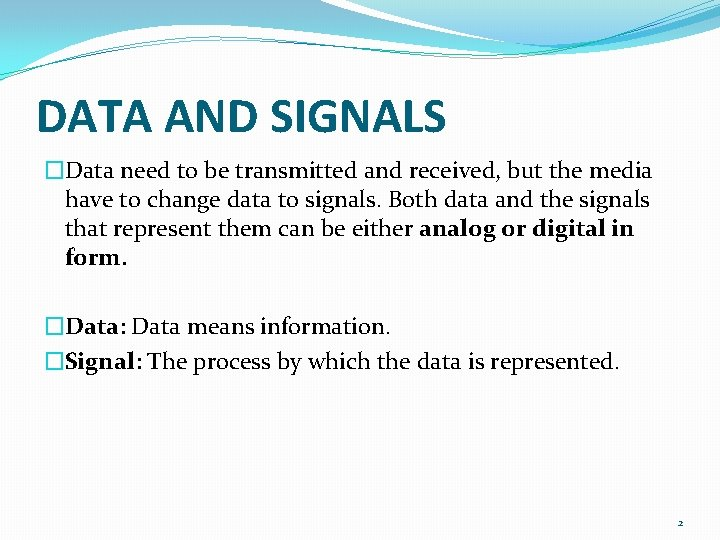DATA AND SIGNALS �Data need to be transmitted and received, but the media have to change data to signals. Both data and the signals that represent them can be either analog or digital in form. �Data: Data means information. �Signal: The process by which the data is represented. 2Analog and Digital Data �Data can be analog or digital. The term analog data refers to information that is continuous; digital data refers to information that has discrete states. For example, an analog clock that has hour, minute, and second hands gives information in a continuous form; the movements of the hands are continuous. On the other hand, a digital clock that reports the hours and the minutes will change suddenly from 8: 05 pm to 8: 06 pm. 3Analog and Digital Data �Analog data, such as the sounds made by a human voice, take on continuous values. When someone speaks, an analog wave is created in the air. This can be captured by a microphone and converted to an analog signal or sampled and converted to a digital signal. �Digital data take on discrete values. For example, data are stored in computer memory in the form of 0 s and 1 s. They can be converted to a digital signal or modulated into an analog signal for transmission across a medium. 4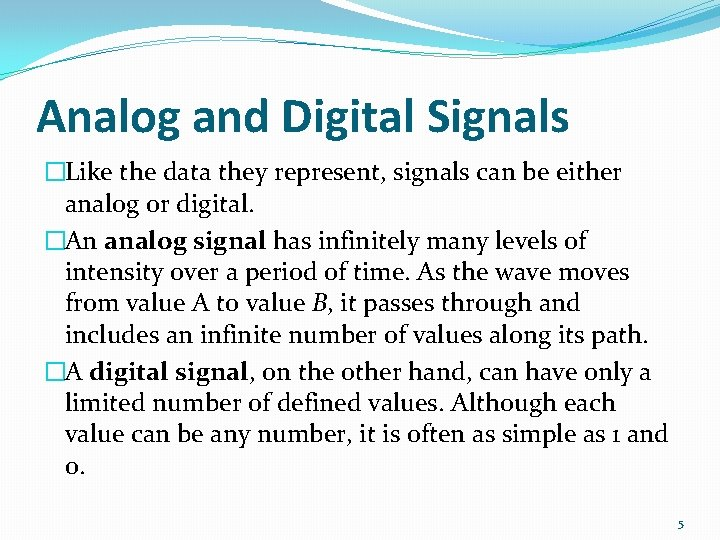Analog and Digital Signals �Like the data they represent, signals can be either analog or digital. �An analog signal has infinitely many levels of intensity over a period of time. As the wave moves from value A to value B, it passes through and includes an infinite number of values along its path. �A digital signal, on the other hand, can have only a limited number of defined values. Although each value can be any number, it is often as simple as 1 and 0. 5Analog and Digital Signals �The simplest way to show signals is by plotting them on a pair of perpendicular axes. The vertical axis represents the value or strength of a signal. The horizontal axis represents time. �Figure below illustrates an analog signal and a digital signal. �The curve representing the analog signal passes through an infinite number of points. The vertical lines of the digital signal, however, demonstrate the sudden jump that the signal makes from value to value. 6Analog and Digital Signals 7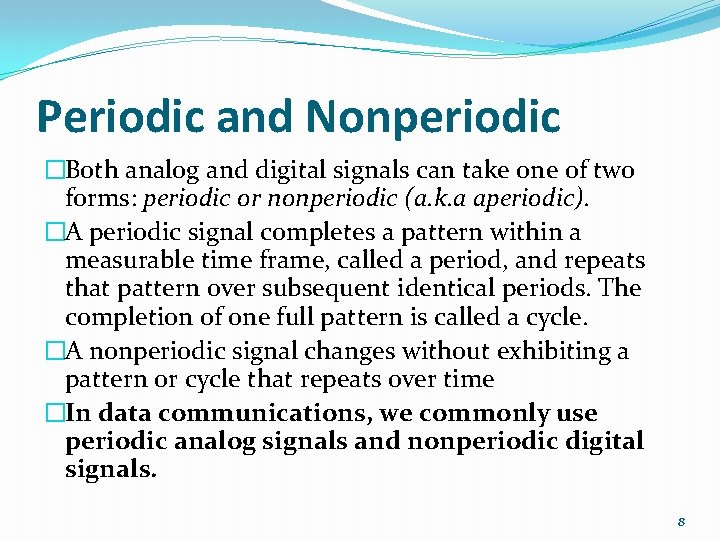Periodic and Nonperiodic �Both analog and digital signals can take one of two forms: periodic or nonperiodic (a. k. a aperiodic). �A periodic signal completes a pattern within a measurable time frame, called a period, and repeats that pattern over subsequent identical periods. The completion of one full pattern is called a cycle. �A nonperiodic signal changes without exhibiting a pattern or cycle that repeats over time �In data communications, we commonly use periodic analog signals and nonperiodic digital signals. 8PERIODIC ANALOG SIGNALS �Periodic analog signals can be classified as simple or composite. �A simple periodic analog signal, a sine wave, cannot be decomposed into simpler signals. �A composite periodic analog signal is composed of multiple sine waves. 9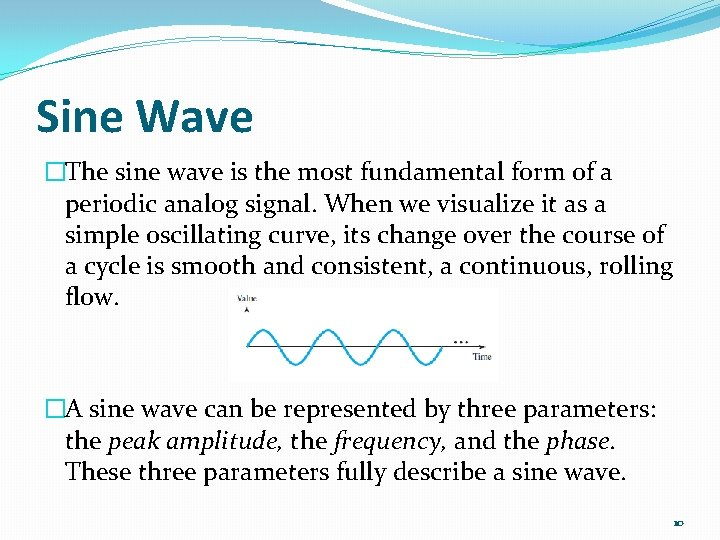Sine Wave �The sine wave is the most fundamental form of a periodic analog signal. When we visualize it as a simple oscillating curve, its change over the course of a cycle is smooth and consistent, a continuous, rolling flow. �A sine wave can be represented by three parameters: the peak amplitude, the frequency, and the phase. These three parameters fully describe a sine wave. 10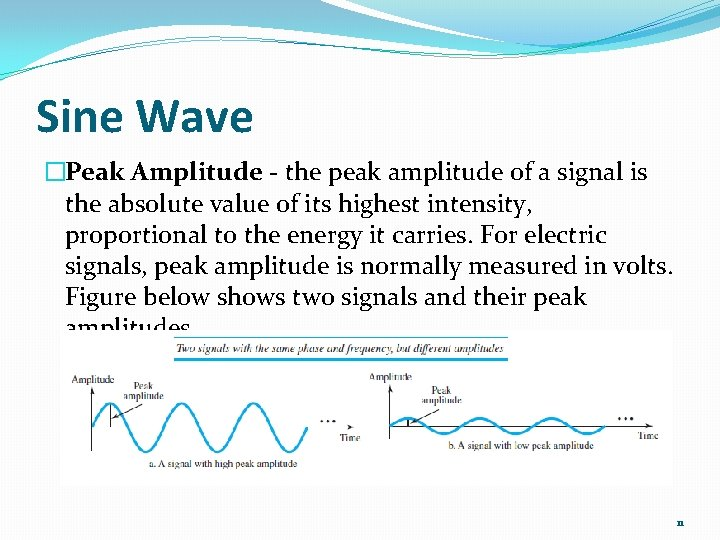Sine Wave �Peak Amplitude - the peak amplitude of a signal is the absolute value of its highest intensity, proportional to the energy it carries. For electric signals, peak amplitude is normally measured in volts. Figure below shows two signals and their peak amplitudes. 11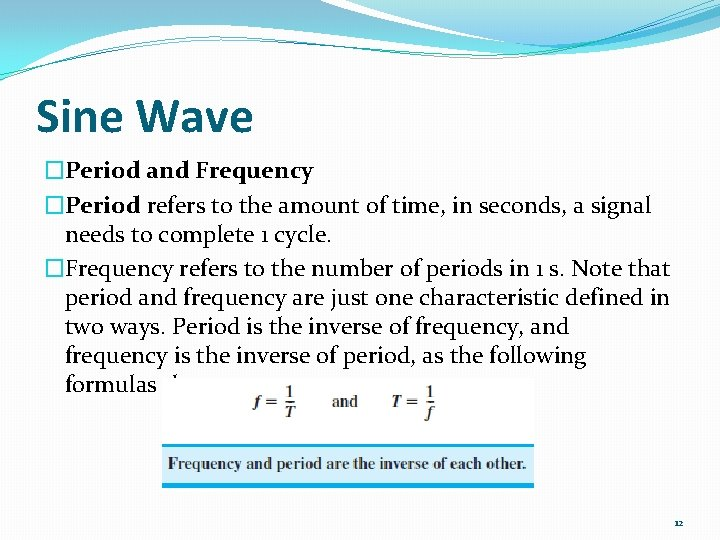Sine Wave �Period and Frequency �Period refers to the amount of time, in seconds, a signal needs to complete 1 cycle. �Frequency refers to the number of periods in 1 s. Note that period and frequency are just one characteristic defined in two ways. Period is the inverse of frequency, and frequency is the inverse of period, as the following formulas show. 12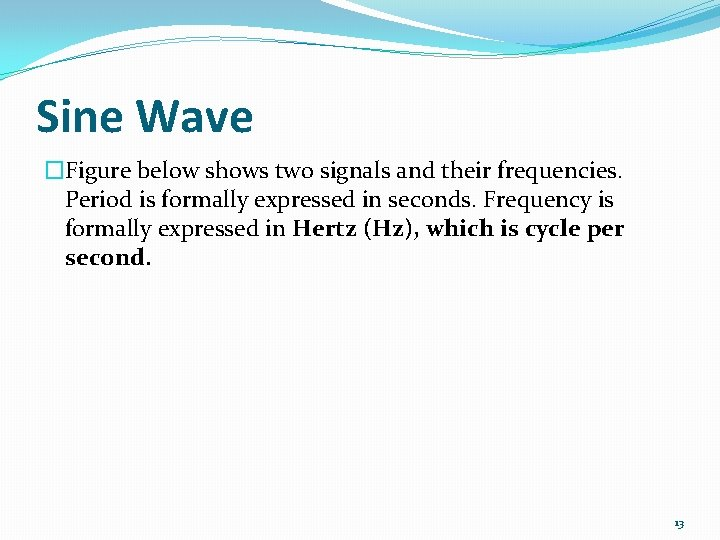Sine Wave �Figure below shows two signals and their frequencies. Period is formally expressed in seconds. Frequency is formally expressed in Hertz (Hz), which is cycle per second. 13Sine Wave 14Sine Wave 15